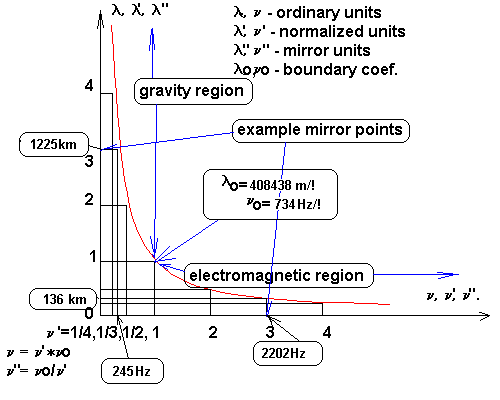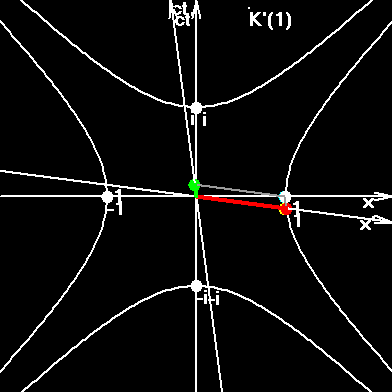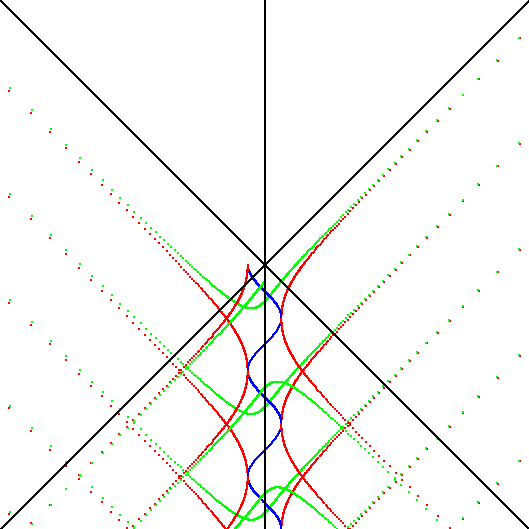# SPACE-TIME SYMMETRY

## The Flash

During the centuries-old history the man has invented a large set of units for measurement of spatial and temporal quantities, but the Nature has its own units. It seems, that in this work the successful choice for transition from artificial units, far-fetched by the man, to natural units was made. Now in physics some types of natural units are already used (for example: nuclear units and relativistic units). In this work, after the postulating of symmetries, the new natural units were received and named here Normalized Unit. Initial postulates were: Equality of coordinates X, Y, Z, T in the microworld and megaworld; Scale symmetry inside and outside from some boundary, that is, the symmetry between X and 1/X relatively 1.

The common unit for measurement of spatial and temporal dimensions was received here, denoted by the sign "!", and named THE FLASH. Thus, 1! = 408438.0458 meters; 1! = 0.001362402672 seconds. Somebody will tell: "There are meters, kilometers, centimeters, inches, seconds, years... Why does the so strange flash necessary?" The answer is simple: "The flash divides the whole scale of fluctuations on two regions: electromagnetic and gravitational."

The last statement we shall decipher in more detail. One flash, as unit of length is approximately equal to 408 kilometers. If the space-time fluctuation has the wavelength more than 408 kilometers, then this is the gravitational fluctuation. Its carrier is graviton. If the space-time fluctuation has the wavelength less than 408 kilometers, then this is the electromagnetic fluctuation. Its carrier is photon. Gravitons form the space-time, the medium in which we exist. Figuratively speaking, the gravitons are seats for passengers. Photons occupy the space-time, that is, photons are not the seats for passengers, but are the passengers themselves.

Space-time, in which we exist, is a huge set of gravitons, oscillators with wavelength more than 408 kilometers. However, this stuff is not so is simple, as it seems at first. Taking into account, that the scale of scales is closed (…small, bigger, bigger… and biggest=smallest, bigger, bigger…), and, that we are multilevel objects, then for each scale level it is possible to find boundary length of fluctuations. That is, for grownup man as a whole the boundary length of space-time fluctuations will be one; for his organs – another; for his cells – the third... There is even an assumption that the boundary wavelength for an adult man and for a newborn child will slightly differ from each other.

Thus, the space-time fluctuation of wavelength, which is equal to 408438.0458 meters in SI units, or to 1 flash in Normalized Units, is the border between photons and gravitons, containing us. The transitive factors between SI and Normalized Units will be: for wavelength - l0 = 408438.0458 m/!; for the period of fluctuations - t0 = 0.001362402672 s/!; for frequency - n0 = 1/t0 = 733.9973861 !/s.

Using the given factors, it is possible to transfer the given value of length, time, and frequency from SI into Normalized Units with the help of the following formulas:

n' = n/n0 (1)

l' = l/l0 (2)

t' = t/t0 (3)

It is interesting to look, what will be behind the border of fluctuations in the symmetrical point for some known fluctuation? In Normalized Units it is done simply: if any fluctuation, for example, with wavelength 8 flashes is known, we search for symmetry in a point with wavelength 1/8 of flash. But flashes, as the units of measurement are unusual, and we can at once transfer the received results into usual units of length and time with the help of the following formulas:

n" = n02/n. (4)

t" = t02/t. (5)

l" = l02/l. (6)

Which can be easily received from the figure:## Symmetry Table

 Object Original value in SI The same value in Normalized Units Mirror results in SI Comparable or equal to: Proton m=1.673*10-27kg n=2.2687*1023Hz l=1.3214*10-15m n'=3.0909*1020 l'=3.2353*10-21 t'=3.2353*10-21 n"=2.3747*10-18Hz l"=1.2625*1026m t"=4.211*1017s n" = H = 73.28km/s/Mpc (Hubble constant). t" = T = 13.34 billion years / rotation, (conventional age of Universe) Electron m=9.11*10-31kg n=1.236*1020Hz l=2.426*10-12m n'=1.68*1017 l'=5.94*10-18 t'=5.94*10-18 n"=4.36*10-15Hz t"=2.29*1014s l"=6.88*1022 m l" = L = 2.23 Mpa. Distances between some groups of galaxies CMBR T=2.728K n=2.82*1011Hz l=1.062*10-3m n'=3.84*108 l'=t'=2.60*10-9 n"=1.91*10-6Hz t"=5.23*105s=6.06days l"=1.57*1014 m 6.06 days ~ Humane week, average period of cepheidas and other classes of stars. Cosmic masers n = 1612 (1665, 1667,1720) MHz n'=2.20*106, (2.27*106, 2.27*106, 2.34*106) l'=t'=4.56*10-7 (4.41*10-7, 4.40*10-7, 4.27*10-7) n"=3.34*10-4 (3.23*10-4, 3.23*10-4, 3.13*10-4) l"=8.97*1011 (9.27*1011, 9.28*1011, 9.58*1011)m l"= L ~ 1 light hour Approximately corresponds to the maser's variability Pulsar NP0531 t = 0.033 s n'=4.12*10-2 l'=t'=24.22 n" = 17.8 kHz l"=16.9 km l" corresponds to pulsar's radius; (n" lies in the region of "whistling atmospherics"). The shorter pulsar's period, the nearer it lies to the boundary region. Earth's effective temperature T=288K l=1.01*10-5m n'=4.05*1010 l'=t'=2.47*10-11 l" =1.65*1016m= 1.75 light years l" ~ 2 earth's years Sun's effective temperature (there exists a big spectrum of temperatures) T=5785K n=5.98*1014Hz l=5.01*10-7m n'=8.14*1011 l'=t'=1.23*10-12 n"=9.01*10-10 l"=3.33*1017 = 35.2 light years Comparable to periods 11 and 22 years Sun's periods of fluctuation 160.01 (there exists a big spectrum of fluctuations) t =160.01min n=1.04*10-4Hz l=2.88*1012m n'=1.42*10-7 l'=t'=7.05*106 l" = 0.0579 m; n" = 5180 MHz Sun's temperature in radio region is almost 106 K 5-minute sun fluctuation period reflects in sun max. radio-region. Temperature of the man (36.6 C) n=3.20*1013 l=9.36*10-6 n'=4.35*1010 l'=t'=2.29*10-11 n"=1.68*10-8Hz l"=1.78*1016m = 1.88 light years *2~the nearest star. Breathing, hartbeet: t=1sec. n=1Hz l=3*108m n'=1.36*10-3 l'=t'=734 n"=539kHz, l"=557m Origin of SI units. Boundary region. Bottom frequency of human's sensible sound n=20Hz n'=0.027 n"=26.9 kHz ~ Upper frequency of human's sensible sound DNA ??? Information . . Earthquakes ??? . and interest . Periodicity in virus pattern ??? . . are needed !

## Quantum of force, and the energy of graviton

The energy of photon, laying in an electromagnetic range of fluctuations is determined thus:

Eph = hn, (7)

The energy of graviton, accordingly, should be determined thus:

Egr = fl, (8.)

where f – the quantum of forces determined from a condition of correctness of both formulas on the border:

E0 = hn0 = hc/l0 = (hc/l02)l0, (9)

f = hc/l02, (10)

In the bottom figure the spectrum of the background microwave radiation and symmetrical spectrum of gravitational radiation are shown.Thus, the quantum of force in the gravitational range of fluctuations corresponds to the quantum of action in an electromagnetic range of fluctuations. Quantum of action works in the microworld. Quantum of force works in the megaworld. The physical sense of quantum of force can be perceived in the following reasoning chain. The universe is at least four-dimensional and closed. Hence, any "straight line" goes on a circumference. The maximal length of a circumference in the universe is X = 1,26245190072E+26 m. The resting proton in three-dimensional space moves in four-dimensional space along a time circumference of the same length. Hence, the concept of centripetal force is applicable to proton: f = ma = mv2/r. Substituting the speed of light instead of velocity, and X instead of r, we shall receive the quantum of force:

f = mc2/X = 1,190760067E-36 N.

If we shall divide not by the large circumference of the universe, but by the radius of curvature of universe, we shall receive fbar, or f*2p , which is the analogue of hbar, or h/(2p). And this will be the minimally possible force applied to any proton in the universe:

fbar =f*2p mc2/r = 7,481766157E-36 N.

In the closed world there is no straight lines; and we can conclude, that any proton is always under the action of the minimal force, determined by the quantum of force, or under the action of the force, which is multiple of quantum of force. Consequently, the force is also the quantum physical quantity.

## Surprising similar results received independently.

In May 2000 I have found out about existence of the work “The Wave Equations for Oscillator with Planck's Constant and with a Quantum of Force”, Phys. Chem. Earth (A), Vol.24, No.8, pp. 721-725, 1999, performed independently by Alexander Malimon. The Internet-link to this work is: http://www.copernicus.org/EGS/pce/part_a_vol_24_no8b.html. The author of the quoted work has received a set of results very close to results received in my work. But instead of "graviton", he uses the term "inverse photon". According to his estimations, the quantum of force lays in a range ~ 10-37 -:- 10-36 N, and the La-mbda point (I name it boundary frequency) lays in the region about 400 Hz. It is also surprisingly that he has assumed the opportunity of existence of low-frequency vacuum fluctuations, symmetric to CMB radiation. According to his estimations, these fluctuations lay in a range from 4 to 40 days. (Compare this result with result in the third line in the table above.) Such "relict radiation" can be expressed in space granulation into cells with the characteristic sizes, which have been mentioned above. A.N. Malimon has assumed, that these cells have their own potentials of gravitational and electromagnetic fields. And these fields can influence on quantum fluctuations of systems in these cells. The author writes about experimental observations of sudden frequency steps, on the clocks based on the quantum frequency standards, which is located in the different parts of the world. In his work Alexander Malimon has written down the equation for harmonic oscillator with the quantum of force. This equation is symmetric to the Schr�dinger equation, which was written down with the quantum of action.

## New results

In the summer of 1999 I have noticed one strange numerical concurrence written in Normalized Units: G' = 1/Exp(a+1/a); where G' - gravitational constant in reducible Normalized Units (units, independent from a humane); a is a fine structure constant. The further analysis has shown, what it must be exactly thus. Indeed, in the very beginning we have imposed the condition of symmetry between gravitational and electromagnetic fluctuations; and a is a constant describing the electromagnetic interactions in the language of quantum electrodynamics. Then G' is the gravitational fine structure constant. An exponent on one hand, or the logarithm on the other hand, occurs as result of the symmetry inside/outside, or the symmetry between X and 1/X. In the summer of 2000 I has received the unexpected development in the analysis of the Graity-Thermal Coil. As a result of this analysis, it was found out, that the Newton gravitation law must be symmetric to Coulomb law. It must contain the gravitational constant in empty space, which is the analogue of electric permittivity of empty space; and relative permittivity of the medium, etc. Look the page The Earth's Core Is Overheated Emptiness for more details. The crazy results, which can be hardly accepted by a scientific public in the future 100 years, are shown on page Gravitational Mirror, where with the help of transformations:

x' = x/(x2+y2), y'=y/(x2+y2);
x = x'/(x'
2+y'2), y=y'/(x'2+y'2), (11)

one can find out the coordinates of our imaginary images. Those transformations are the elementary transformations inside/outside; the transformations of photon space into graviton space and visa verse. These transformations will be useful in geology, because they connect our real and imaginary images, relatively the border between the mantle and the Earth’s core; and also in biology, relatively the membrane of a cell, with a DNA-molecule on one hand of transformations, and the humane, carrier of this molecule, on the other hand of transformation equations. (The DNA-molecule is a reflection of a human, who lives or lived somewhere in the far end of our closed but boundless Universe.)

To index of Space Genetics

Ivan Gorelik.

My Curriculum Vitae.

My VB-program SR2007.exe proves that the electron is not a point, but a string, embracing the whole Universe in a period, equal to electron's classic period.Particles sew and stitch the space-time, constantly recharging electric and colour field, which are constituent subspaces of our whole macroscopic space-time.Interested? Then go to the index of Space Genetics